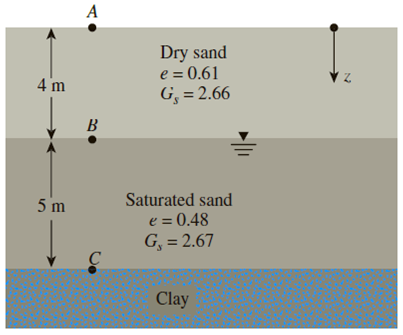Chapter 9, Problem 9.5PPrinciples of Geotechnical Enginee...

9th Edition
Braja M. Das + 1 other
ISBN: 9781305970939

Solutions

Chapter
SectionPrinciples of Geotechnical Enginee...

9th Edition
Braja M. Das + 1 other
ISBN: 9781305970939
Textbook Problem

Consider the soil profile shown in Figure 9.26:a. Calculate the variations of σ, u, and σ′ at points A, B, and C.b. How high should the groundwater table rise so that the effective stress at C is 111 kN/m2?Figure 9.26

a)

To determine

Find the variation of total stress σ, pore water pressure (u), and the effective stress σ at point A, B, and C.

Explanation

Given information:

The thickness H1 of dry sand layer 1 is 4.0 m.

The specific gravity Gs of the dry sand is 2.66.

The void ratio (e) of the dry sand is 0.61.

The thickness H2 of saturated sand layer 2 is 5.0 m.

The specific gravity Gs of the saturated sand is 2.67.

The void ratio (e) of the saturated sand is 0.48.

Calculation:

Determine the dry unit weight γd of the sand in the first layer using the relation.

γd=Gsγw1+e

Here, γw is the unit weight of the water.

Take the unit weight of the water as 9.81kN/m3.

Substitute 2.66 for Gs, 9.81kN/m3 for γw, and 0.61 for e.

γd=2.66×9.811+0.61=16.2kN/m3

Determine the saturated unit weight γsat(layer2) of the saturated sand in the second layer using the relation.

γsat(layer2)=(Gs+e)γw1+e

Substitute 2.67 for Gs, 9.81kN/m3 for γw, and 0.48 for e.

γsat(layer2)=(2.67+0.48)×9.811+0.48=20.88kN/m3

Calculate the total stress at point A using the relation.

σ=0

Thus, the total stress at point A is zero_.

Calculate the pore water pressure at point A using the relation.

u=0

Thus, pore water pressure at point A is zero_.

Calculate the effective stress at point A using the relation.

σ=σu

Substitute 0 for σ and 0 for u.

σ=00=0

Thus, effective stress at point A is zero_.

Calculate the total stress at point B using the relation.

σ=γd×H1

Substitute 16.2kN/m3 for γd and 4.0 m for H1.

σ=16.2×4.0=64

b)

To determine

Find the rise of water table to increase the effective stress at point C is equal to 111kN/m2

Still sussing out bartleby?

Check out a sample textbook solution.

See a sample solution

The Solution to Your Study Problems

Bartleby provides explanations to thousands of textbook problems written by our experts, many with advanced degrees!

Get Started

How is knurling different from other lathe operations?

Precision Machining Technology (MindTap Course List)

What is data independence, and why is it lacking in file systems?

Database Systems: Design, Implementation, & Management

Define the term topology, and draw a sketch of each wired and wireless network topology.

Systems Analysis and Design (Shelly Cashman Series) (MindTap Course List)

What two welding processes are semiautomatic processes?

Welding: Principles and Applications (MindTap Course List)

What Internet access plans are available in your area?

Enhanced Discovering Computers 2017 (Shelly Cashman Series) (MindTap Course List)